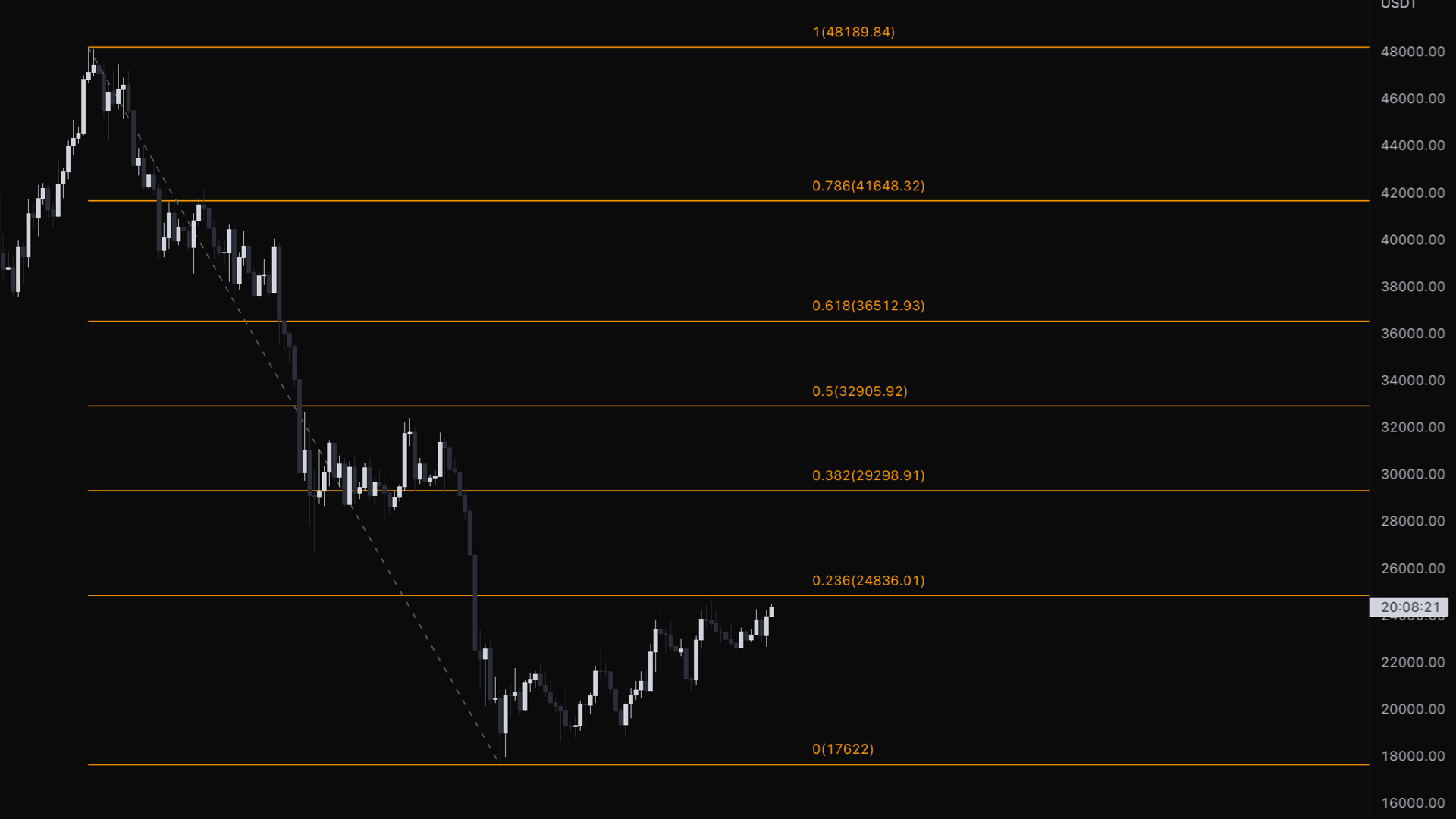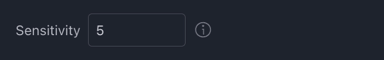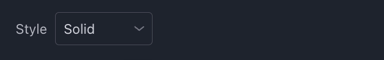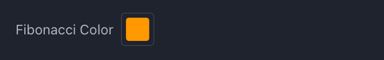# FibonacciFibonacci Retracements use the Fibonacci Retracement levels of 0, .236, .382, .5, .618, .786, and 1.

This indicator will Automatically pull a Fibonacci Retracement from a previous low to high swing or high to low swing based on the direction of the Price.

Each level is associated with a percentage. The percentage is how much of a prior move the price has retraced. The Fibonacci retracement levels are 23.6%, 38.2%, 61.8%, and 78.6%. While not officially a Fibonacci ratio, 50%, 0%, and 100% is also used.

Fibonacci retracement levels connect any two points that the trader views as relevant, typically a high point and a low point. These points or as we call them “Swings”. If the Price goes from a low swing to a high swing then the indicator will pull the Fibonacci Retracement from the low swing to the high swing and the retracement is the Price Action that retraces back to the low swing. If the Price goes from a high swing to a low swing then the indicator will pull the Fibonacci Retracement from the high swing to the low swing and the retracement is the Price Action that retraces back to the high swing.

The percentage levels provided are areas where the price could stall or reverse. The most important levels are .5 or 50% and .618 or 61.8%.

The most commonly used ratios include 23.6%, 38.2%, 50%, 61.8%, and 78.6%.

These levels should not be relied on exclusively, so it is dangerous to assume the price will reverse after hitting a specific Fibonacci level. Therefore it is better to use Fibonacci Retracements as points of Confluence with other Key Levels.

Fibonacci Retracement Modes:

Off - The default position and turns this tool off

Regular - Tool will plot a normal Fibonacci Retracement

Reverse - If the indicator plots a Fibonacci Retracement that is in the wrong direction then plotting it in the Reverse will flip the Retracement into the correct direction.

# Sensitivity

This is related to the Sensitivity of the Fibonacci Retracements and changes the lookback length and deviation of the indicator

The default setting is 5 and has a sensitivity range of 1 to 10 at .5 increments.

Increasing the sensitivity will plot the Fibonacci Retracements closer to the current Price Action. Decreasing the sensitivity will plot the Fibonacci Retracements further away from the current Price Action.# StyleAdjusts the type of lines used by the Fibonacci Retracement. The default line is solid but can be changed to dotted or dashed

# ColorThe default color is orange but can be changed to any color.